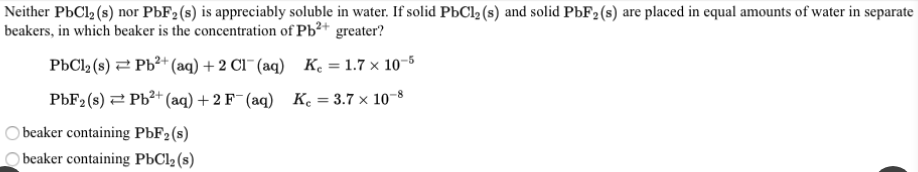# Neither PbCl2(2) nor PbF2(s) is appreciably soluble in water. If solid PbCl2(s) and solid PbF2(s) are placed in equal amounts of water in separate beakers, in which beaker is the concentration of Pb^2+ greater? PbCl2(s) ⇌ Pb^2+(aq) + 2Cl^-(aq) Kc = 1.7X10^-5 PbF2(s) ⇌ Pb^2+(aq) + 2 F^-(aq) Kc = 3.7x10^-8